Courses

# Low And High Cycle Fatigue Mechanical Engineering Notes | EduRev

## Mechanical Engineering : Low And High Cycle Fatigue Mechanical Engineering Notes | EduRev

The document Low And High Cycle Fatigue Mechanical Engineering Notes | EduRev is a part of the Mechanical Engineering Course Machine Design.
All you need of Mechanical Engineering at this link: Mechanical Engineering

Low cycle fatigue

This is mainly applicable for short-lived devices where very large overloads may occur at low cycles. Typical examples include the elements of control systems in mechanical devices. A fatigue failure mostly begins at a local discontinuity and when the stress at the discontinuity exceeds elastic limit there is plastic strain. The cyclic plastic strain is responsible for crack propagation and fracture. Experiments have been carried out with reversed loading and the true stressstrain hysteresis loops are shown in figure-3.4.1.1. Due to cyclic strain the elastic limit increases for annealed steel and decreases for cold drawn steel. Low cycle fatigue is investigated in terms of cyclic strain. For this purpose we consider a typical plot of strain amplitude versus number of stress reversals to fail for steel as shown in figure-3.4.1.2.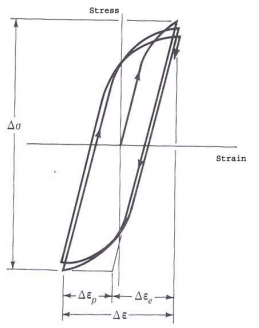3.4.1.1F- A typical stress-strain plot with a number of stress reversals .

Here the stress range is Δσ. Δεp and Δεe are the plastic and elastic strain ranges, the total strain range being Δε. Considering that the total strain amplitude can be given as

Δε =  Δε + Δε

A relationship between strain and a number of stress reversals can be given as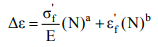where σf and εf are the true stress and strain corresponding to fracture in one cycle and a, b are systems constants. The equations have been simplified as follows: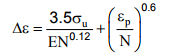In this form the equation can be readily used since σu, εand E can be measured in a typical tensile test. However, in the presence of notches and cracks determination of total strain is difficult.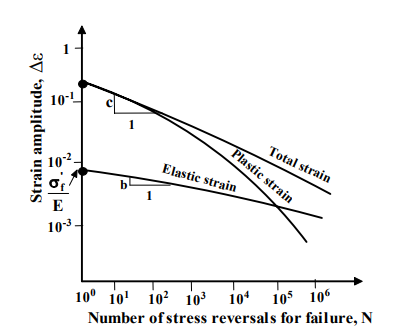3.4.1.2F- Plots of strain amplitude vs number of stress reversals for failure.

High cycle fatigue with finite life

This applies to most commonly used machine parts and this can be analyzed by idealizing the S-N curve for, say, steel, as shown in figure- 3.4.2.1 .
The line between 103 and 106 cycles is taken to represent high cycle fatigue with finite life and this can be given by

logS =  blogN +c

where S is the reversed stress and b and c are constants.

At point A log (0.8σu) =  blog103  + c where σu is the ultimate tensile stress

and at point Blog σc = blog106 + c where σe is the endurance limit.

This gives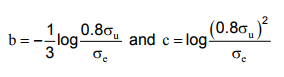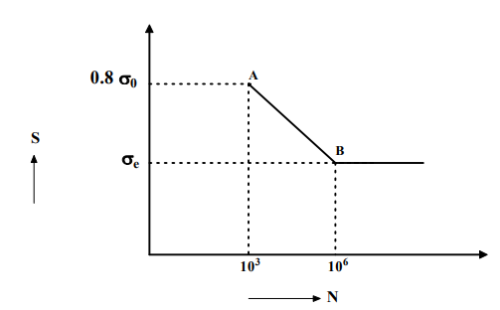3.4.2.1F- A schematic plot of reversed stress against number of cycles to fail.

Fatigue strength formulations

Fatigue strength experiments have been carried out over a wide range of stress variations in both tension and compression and a typical plot is shown in figure3.4.3.1. Based on these results mainly, Gerber proposed a parabolic correlation and this is given by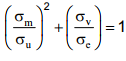Gerber line

Goodman approximated a linear variation and this is given by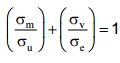Goodman line

Soderberg proposed a linear variation based on tensile yield strength σY and this is given by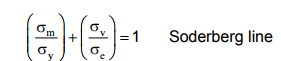Here, σand σv represent the mean and fluctuating components respectively.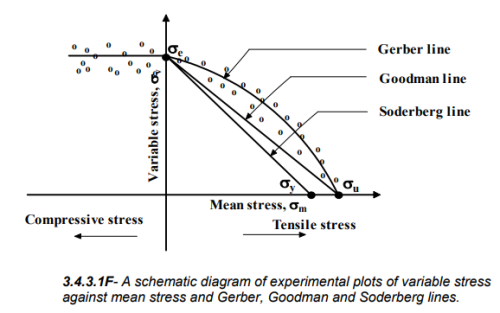Offer running on EduRev: Apply code STAYHOME200 to get INR 200 off on our premium plan EduRev Infinity!

## Machine Design

57 videos|71 docs|102 tests

,

,

,

,

,

,

,

,

,

,

,

,

,

,

,

,

,

,

,

,

,

;